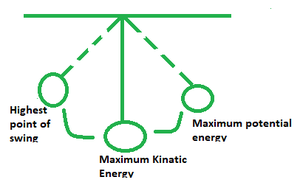# Oscillatory Motion Formula

• Last Updated : 26 Apr, 2022

Oscillatory Motion is a form of motion in which an item travels over a spot repeatedly. The optimum situation can be attained in a total vacuum since there will be no air to halt the item in oscillatory motion friction.

Let’s look at a pendulum as shown below.The vibrating of strings and the movement of spring are both oscillatory motions in the mechanical world and are the same as mechanical vibration. The periodic motion should not be confused with oscillatory motion. Objects in periodic movements repeat their motion after a set amount of time, whereas objects in oscillatory motions repeat their motion over a defined position.

### Hooke’s Law

Basic Harmonic Motion is a simple type of oscillatory motion (SHM). The restoring force in this motion is proportional to the displacement from the equilibrium position. This is known as Hooke’s Law.

Example

Assume a mass m block is linked at one end with a long spring (spring constant k) and the other end is anchored to the wall. Hooke’s law expresses the restoration force that attempts to repair the deformation of a spring.

Formula

T =where,

• l denotes the length of the pendulum
• g denotes the acceleration due to gravity

### Sample Problems

Problem 1. Find the length of a pendulum with a period of 0.7 s.

Solution:

The period of a pendulum of length l = T =⇒L = 0.121 m

Problem 2. Assuming g is constant, when does a simple pendulum double its period?

Solution:If the length were to be made four times the original, the period becomes= 2T

Hence you need to make the length four times its original value in order to achieve double period, given g is constant.

Problem 3. Can friction be restoring force for oscillations?

Solution:

Friction acts opposite to the direction of motion. As the item is pushed away from its mean position, the spring’s force varies, acting as a restoring force. Yes friction can act as a restoring force.

Problem 4. Are all oscillations curvilinear?

Solution:

Every oscillating movement component replicates its location after a fixed length of time, hence every oscillatory motion is periodic, and not curvilinear.

Problem 5. What do you mean by equilibrium point?

Solution:

In oscillatory motion, an item travels in such a way that it periodically comes to and leaves a fixed point. The equilibrium point is this fixed position.

My Personal Notes arrow_drop_up
Recommended Articles
Page :